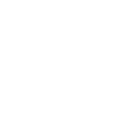×7th Chapter

### 12th Class Mathematics Chapter 7 Test

Here you can prepare 12th Class Mathematics Chapter 7 Vectors Test. Click the button for 100% free full practice test.

## 12th class Mathematics chapter 7 online mcq test with answers for Fa part 2 Mathematics Chapter 7 (Vectors)

This online test contains MCQs about following topics:.

- Introduction - Introduction of Vector in Space - The Scalar Product of Two Vectors - Cross Product or Vector Product of Two Vectors - Scalar Triple Product of Vectors

FA Part 2 Mathematics Ch 7 Test### 12th class Mathematics chapter 7 online mcq test with answers for Fa part 2 Mathematics Chapter 7 (Vectors)

1 <u>i</u>.<u>(j</u>.<u>k)</u> =
• A. Meaningless
• B. -1
• C. 1
• D. 2
2 Zero vector is perpendicular to:
• A. Every vector
• B. Unit vector only
• C. Position vector only
• D. Not any vector
3
• A. Scalar
• B. Free vector
• C. Unit vector
• D. Null vector
4 Which are the following triples can be direction angles of a single vector:
• A. 45°,45°,60°
• B. 30°,45°,60°
• C. 45°,60°,60°
• D. 30°,30°,30°
5 Which of the following is a vector quantity ?
• A. Work
• B. Temperature
• C. Distance
• D. Displacement
6 A scalar quantity is one that possesses only :
• A. Magnitude
• B. Direction
• C. Both a and b
• D. None of these
7
• A. 0
• B.
• C.
• D.
8
• A. 0
• B. 2
• C. 3
• D. 1
9
• A. Unit vector
• B. Null vector
• C. Position vector
• D. None of these
10
• A. 
• B. [0, 0]
• C. [0, 0, 0]
• D. None of these

Sort By:
X

to continue to ilmkidunya.comFill the form. Our admission consultants will call you with admission options.

X

to continue to ilmkidunya.com

X

to continue to ilmkidunya.com

X